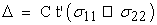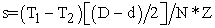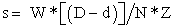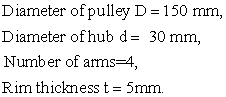﻿ Effect of Rim Thickness on Load Sharing in the Rotating Elements

### Effect of Rim Thickness on Load Sharing in the Rotating ElementsOPEN ACCESSPEER-REVIEWED

## Effect of Rim Thickness on Load Sharing in the Rotating Elements

Rashmi UddanwadikerDepartment of Mechanical Engineering, Visvesvaraya National Institute of Technology, Nagpur, India

### Abstract

The aim of the study is to obtain an optimal arm section for the structures like pulleys, gears, flywheels etc. considering variation of rim thickness with pulley radius. Presently, the design of arm pulley with thin rim is being carried out with an assumption that the bending moment (BM) is shared by half the number of arms, whereas in case of arm gear or V-Belt pulley the assumption is made that BM is shared by total number of arms. In the present paper, Finite Element Method (FEM) is used to determine the BM shared by the arms for its different angular positions. The distribution of bending stress in the arms is also obtained. Finally, design modifications have been suggested considering radius to rim thickness ratio (r/t). Hence it is concluded that when, 15 ≥ r/t > 10.5 – BM is shared by N/2 number of arms, 10.5 ≥ r/t > 7.5 – BM is shared by 4N/7 number of arms, 7.5 ≥ r/t > 5 – BM is shared by 2N/3 number of arms, 5 ≥ r/t > 3 – BM is shared by 4N/5 number of arms, 3 ≥ r/t –BM is shared by N number of arms.

### At a glance: Figures

12
Prev Next

• Uddanwadiker, Rashmi. "Effect of Rim Thickness on Load Sharing in the Rotating Elements." American Journal of Mechanical Engineering 1.5 (2013): 126-130.
• Uddanwadiker, R. (2013). Effect of Rim Thickness on Load Sharing in the Rotating Elements. American Journal of Mechanical Engineering, 1(5), 126-130.
• Uddanwadiker, Rashmi. "Effect of Rim Thickness on Load Sharing in the Rotating Elements." American Journal of Mechanical Engineering 1, no. 5 (2013): 126-130.

 Import into BibTeX Import into EndNote Import into RefMan Import into RefWorks

### 1. Introduction

Arms of the structures like pulleys, gears and flywheel are mainly subjected to bending stresses. It is observed that load shared by individual arm depends on its angular position with respect to the load direction. The stresses induced also depend on the rim thickness. For very small rim thickness, like flat belt pulley, stresses induced are very high, as rim side end of the arm is like free end. The arm in this case behaves like cantilever beam fixed at one end. Present design practice for this case is to assume that half of the arms share the total bending moment (BM). For very large rim thickness like gear the arm behaves like beam fixed at both the ends and hence subjected to lower bending stresses. In such cases therefore, it is assumed that total number of arms share the BM [1-6].

However, these assumptions are based on qualitative analysis regarding how BM is shared, for varying thickness of the rim. The quantitative analysis regarding how load and therefore BM is shared by the arms for varying rim thickness is not reported in the literature. The present research work is therefore aimed at studying the effect of rim thickness and length of the arm on load shared by different arms in the rotating elements.

The analysis is carried out in two ways: (i) Photo elasticity theory (ii) Finite element stress analysis of 2D model for verification of results. The results of stress analysis are obtained with photo elasticity with some specific rim thickness and the finite element procedure on a virtual model similar to the photo-elastic epoxy model is used for verification of the results. Analysis on a virtual model with various rim thickness in equal interval has been carried out. Data is collected for different values of radius to rim thickness ratio (r/t ratio) at various loading positions.

### 2. Photoelasticity

The experimentation is conducted using the concept of photo elasticity. The concept is used to determine stress distribution and stress concentration factors in irregular geometries. The method is based on the property of birefringence, which is exhibited by certain transparent materials. Birefringence is a property by virtue of which a ray of light passing through a birefringent material experiences two refractive indices . Thus, a pulley model made out of such material is selected for the study, to ensure that the state of stress in the model is similar to that of the structure.

2.1. Stress Optic Law

When plane polarized light passes through a photo elastic material, it resolves along the two principal stress directions and each of these components experiences different refractive indices. The difference in the refractive indices leads to a relative phase retardation between the two component waves. The magnitude of the relative retardation is given by the stress optic law:(1)

Where ∆ is the induced retardation in mm, C is the stress optic coefficient in mm2/N, t’ is the specimen thickness in mm, σ11 and σ22 is the first and second principal stress in N/mm2 respectively.

The two waves then pass through wave plates and analyzer resulting into isochromatic and isoclinic fringe patterns. Thus, the state of stress at various points in the material can be determined by these fringe patterns.

Photo elastic sheet has been prepared by mixing CY-230 epoxy resin and HY-951 hardener, 10.5% by volume.

The circular disc is cut and calibration is done to find the material fringe value fσ. As shown in Figure 1 various fringes are obtained for different values of load and finally from the readings it is found that material has fσ as 10.857 N/mm-fringe.

2.2. Experimental Analysis of Pulley Model

The experimental model of pulley with 4 arms has been cut from same photoelastic material sheet. The loading conditions are simulated and pulley is loaded as shown in Figure 2. The figure also shows the stresses developed in the arms and the rim of the pulley. The experiments are carried out for pulleys having different rim thickness and results are given in Table 1.

### 3. Analytical Procedure

Conventionally while designing the arm pulley where rim thickness is very small it is considered that load is shared by only half the number of arms [6, 8]. It is assumed that one end of the arm is fixed at hub and other end of the arm is similar to free end. So the maximum bending stress on the arm of the pulley is given as:(2)

Where, N = n/2, where n is number of arms.

Where, T1 & T2 are belt tensions in tight and slack side respectively. D & d are pulley diameter & hub diameter respectively and N & Z are number of arms sharing bending moment and section modulus of arm near the hub respectively. In the present case for D = 150 mm, d = 30 mm, n = 4, (T1 – T2) = 58.86 N, hence the stress induced comes to be 10.4485 N/mm2.

Whereas, in case of arm gear design where rim thickness is sufficiently large it is considered that the load is equally shared by total number of arms. So the bending stress  on the arm of the pulley is given as(3)

Where, N = n, W is the stalling load, D & d are pulley diameter & hub diameter respectively and N & Z are number of arms sharing bending moment and section modulus of arm near the hub. In the present case, the analytical value is calculated as 5.2242 N/mm2.

### 4. Finite Element Analysis (FEA)

Finite element method [9, 10] has become a powerful tool for numerical solution of a wide range of engineering problems. For the stress analysis, a model of armed wheel has been prepared in ANSYS Workbench version 14 as shown in Figure 3. Plane 183 element has been considered for the FE analysis. The arms are designated as arm number 1, 2, 3 & 4 as shown in Figure 3 (a). The major dimensions of pulley considered for analyses are as follow:Figure 3. (a) 3 dimensional CAD model of an arm pulley showing four arms (b) Normal stress distribution on the arm pulley model along Y-axis

Further, rim thickness has been modified according to the analysis. The load and constraint have been applied according to the actual operating conditions of the pulley. The FE analysis is then carried out to determine the dominating bending stresses in the arms and rim. The FE analysis has been carried out for different (r/t) ratio and different angular locations of the arms. As an illustration, Figure 3 (b) shows the distribution of the stresses in the arm and the rim for one of the situations.

#### Table 2. Bending stress in N/mm2 on arm at different loading angle

4.1. FEA for Different Rim Thickness

FE analysis is carried out by considering different rim thickness and by keeping the major dimensions same. The maximum bending stress on each arm is calculated for rim thickness 5, 10, 15 & 20 mm as shown in Table 1 and its graphical representation is shown in Figure 4 (a).

4.2. FEA for Different Angular Positions of Arm

To check for the worst condition, FE analysis is carried out by changing the locations of arm in anticlockwise direction for each thickness of the arm. The increment in angular position of loading is done in step of 10° from 0° to 80°. The stresses obtained on either of arm no. 1 or 4 are shown in Table 2 and represented graphically in Figure 4 (b).

Figure 4. (a) Maximum Bending stress on each arm for different rim thickness (b) Variation of Bending stress at different rim loading angle

### 5. Results & Discussion

The results of experimental study and FE analysis are compared to verify the correctness of the procedure. The results are given in Table 1 and Table 3 respectively. The results of FEA and experimental are in good agreement for all the cases accept when rim thickness is very small i.e. 5 mm. This may be due to load and constraint which could not be applied realistically due to very thin rim. It has been observed that stresses induced in the arms are reducing as the rim thickness increases.

For the pulley having rim thickness 25mm, FE and conventional approach calculates stress induced as 5.3538 MPa and 5.2242 MPa respectively. This indicates that assumptions made in conventional approach that all the arms equally share bending moment when rim thickness is sufficiently high is quite satisfactory.

From the previous section it is observed that as the rim thickness of the pulley increases the stress on the arms gets equally distributed. For the case of 25mm rim thickness pulley, the stresses on each arm are nearly equal.

The absolute rim thickness does not give idea about rigidity with which arm is fixed to the rim, as it depends upon radius of pulley also. Hence, instead of absolute values of thickness, the ratio (r/t) is varied and stresses induced in the arms are calculated as shown in Table 4 and the graphical representation as shown in Figure 5.

The stress variation given in the table indicates that as the ratio increases the stress induced in the arm reduces. If the stresses obtained by FEA are compared with conventional analysis as given in equation 2 and 3 the number of arms sharing bending moment can be calculated for different range of (r/t) ratio. The maximum bending stresses for 5 mm rim thickness pulley by FEA is shown in Figure 6 (a).

From, Table 4 it is concluded that when, 15 ≥ r/t > 10.5 – BM is shared by N/2 number of arms, 10.5 ≥ r/t > 7.5 – BM is shared by 4N/7 number of arms, 7.5 ≥ r/t > 5 – BM is shared by 2N/3 number of arms, 5 ≥ r/t > 3 – BM is shared by 4N/5 number of arms, 3 ≥ r/t –BM is shared by N number of arms.

### 6. Optimal Design of Arms

This analysis is carried out to design the optimal section of the arms of rotating elements. The design is carried out by conventional method for arm pulley considering that the arm is acting like cantilever beam and load is shared by half the number of arms. The rectangular cross section of arm obtained for 5 mm rim thickness pulley is 14mm x 7mm for maximum stress acting as 15 N/mm2. FE analysis is carried out to find out the stresses acting on arm, the stress obtained is to be 14.3 N/mm2 which is in good agreement, which is shown in Figure 6 (b).

Similarly design is carried out considering that BM is shared by all the numbers of arms for larger rim thickness and that the arm is considered as fixed at both the ends. The rectangular cross section of arms obtained is 11mm x 5.5mm for maximum stress acting as 15 N/mm2. FE analysis is carried out to find out the stresses acting on arm, the stress obtained is to be 14.426 N/mm2 which is in good agreement, which is shown in Figure 6 (c).

Now for the rim thickness which is in between these two conditions, say 10 mm, the design will be carried out by these two conventional processes. The cross section obtained will be same as described earlier. FE analysis is carried out on the rim thickness of 10 mm and with both the arm cross section as shown in Figure 6 (d) and Figure 6 (e).

It can be seen from Figure 6 (d) that the stress induced in the arm for the cross section obtained from considering half the number of arms share load is 10.072 N/mm2, which is very less i.e. the arm section is bigger or the design is bulky. Similarly, the stresses acting on arm for the cross section obtained from considering all the number of arms shared the load is 17.029 N/mm2, which is larger than maximum value i.e. the design is unsafe.

Hence, in such case the analysis explained above is useful to get the optimum section of the arm. So the design is optimized by result obtained from the previous section. The cross section obtained by considering 2N/3 number of arms shared the load is 12.5 mm x 6.25 mm. FE analysis carried out is shown in Figure 6 (f) which shows that the stresses acting on the arm is 14.759 N/mm2, which is the optimized stress, close to the maximum stress. So the design is considered as the optimized design.

Figure 6. (a) Maximum Bending stresses for 5mm rim thickness pulley (b) Stress acting on arm for small rim thickness.(c) Stress acting on arm for larger rim thickness. (d)Stress acting on arm with bigger cross section. (e) Stress acting on arm with smaller cross section. (f) Stress acting on arm with optimized cross section

### 7. Conclusion

For very thin rim thickness (r/t > 10.5) it has been validated that the load is shared by N/2 numbers of arms. As the rim thickness increases stresses converge and get equally distributed in the arms. The rim become more rigid, so arm can be considered as fixed on both side instead of cantilever support. The consideration that half the number of arms share load no longer holds true. For the ratio (r/t ≤ 3) it can be assumed that all the arms share the BM. However for the r/t ratios between 3 and 10.5, it is desirable to find out how many arms share the BM to get optimum section of the arms. In the present study FEA and photo elasticity approaches are used to determine this number. Hence it is concluded that when, 15 ≥ r/t > 10.5 – BM is shared by N/2 number of arms, 10.5 ≥ r/t > 7.5 – BM is shared by 4N/7 number of arms, 7.5 ≥ r/t > 5 – BM is shared by 2N/3 number of arms, 5 ≥ r/t > 3 – BM is shared by 4N/5 number of arms, 3 ≥ r/t –BM is shared by N number of arms.

### Acknowledgement

Author wishes to thank Dr. D.V. Bhope for his valuable suggestions and also wish to thank Mr. Himanshu Authankar and Mr. R. Giripunje for helping in cutting experimental pulley model.

### References

  Shigley J.E. “Mechanical engineering design.” 5th Edition, McGraw-Hill International, 1986.In article  L.M. Valadimin, J.B. Hartman, “Machine Design”, CBS Publisher, Delhi, 1983.In article  Peter R. N. Childs “Mechanical Design” 2nd Edition, Elsevier Butterworth-Heinemann publication, 2004.In article  PSG College of Technology “Design Data”, Kalaikathir Achchagam, 1978.In article  K. Lingaiyah, “Machine Design Handbook”, McGraw-Hill, New York, 1994.In article  Shigley J.E, Charles R. Mischke, Thomas H. Brown, Jr. “Standard Handbook Of Machine Design” 3rd Edition, McGraw-Hill International, 2004.In article  J.W.Dally & W.F.Riley, “Experimental stress analysis”, Springer Publisher, 1993.In article PubMed  N. W. Pathan, Dr. D.V. Bhope, Prof. S.D Khamankar “Investigation of stresses in flat belt pulley by fem and photoelasticity”. Oct 2011.In article  S.S. Bhavikatti, “Finite Element Analysis” New Age International, 2007.In article  P. Seshu, “Textbook of Finite Element Analysis”. PHI Learning Pvt. Ltd., 2004.In article PubMed